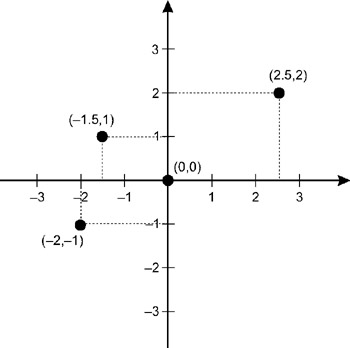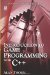# 3.2 2D Coordinates

## 3.2 2D Coordinates

Extending from the single number line idea, a 2D coordinate system can be created. Two number lines-one horizontal and one vertical-intersect. The place where they intersect is called the origin since it is the point of 0 on both lines. The horizontal line is x (like in algebra) and the vertical is y. x, as already seen, measures distances from left to right along the standard number line, and y measures distances in the same way, but up and down instead of left to right. So, using any ordered pair of numbers like (3, 2) means any point can be expressed from the origin within the boundaries of the two lines, as shown in Figure 3.2. The order of the pair is in the form of (x, y), so 3 represents the distance to travel along x, and 2 is the distance to travel along y. This form of coordinate system is called a Cartesian coordinate system, named after the French mathematician and philosopher René Descartes. The ordered pair of numbers expressing a point within the coordinate space is called the Cartesian coordinates, or simply the coordinates. The 2D coordinate system is also called a plane, just as the 1D system can be thought of as a line. So, any point in a 2D coordinate system can be called a point on a plane.Figure 3.2: 2D coordinatesIntroduction to Game Programming with C++ (Wordware Game Developers Library)
ISBN: 1598220322
EAN: 2147483647
Year: 2007
Pages: 225
Authors: Alan Thorn

Similar book on Amazon

flylib.com © 2008-2017.
If you may any questions please contact us: flylib@qtcs.net